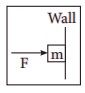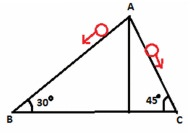# UNIT III: Laws of motion - Online Test

Q1. When a car takes a sudden left turn in the curved road, passengers are pushed towards the right due to
Explaination / Solution:
No Explaination.

Q2. An object of mass m held against a vertical wall by applying horizontal force F as shown in the figure. The minimum value of the force F isExplaination / Solution:
No Explaination.

Q3. A vehicle is moving along the positive x direction, if sudden brake is applied, then
Explaination / Solution:
No Explaination.

Q4. A book is at rest on the table which exerts a normal force on the book. If this force is considered as reaction force, what is the action force according to Newton’s third law?
Explaination / Solution:
No Explaination.

Q5. Two masses m1 and m2 are experiencing the same force where m1< m2. The ratio of their acceleration a1/a2 is
Explaination / Solution:
No Explaination.

Q6. Choose appropriate free body diagram for the particle experiencing net acceleration along negative y direction. (Each arrow mark represents the force acting on the system).
Explaination / Solution:
No Explaination.

Q7. A particle of mass m sliding on the smooth double inclined plane (shown in figure) will experienceExplaination / Solution:
No Explaination.

Q8. Force acting on the particle moving with constant speed is
Explaination / Solution:
No Explaination.

Q9. An object of mass m begins to move on the plane inclined at an angle θ. The coefficient of static friction of inclined surface is μs. The maximum static friction experienced by the mass is
Explaination / Solution:
No Explaination.

Q10. When the object is moving at constant velocity on the rough surface,
Explaination / Solution:
No Explaination.